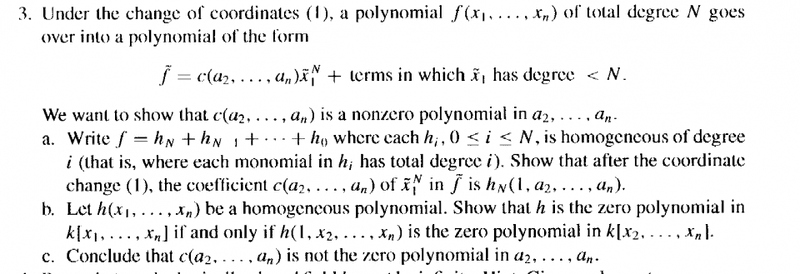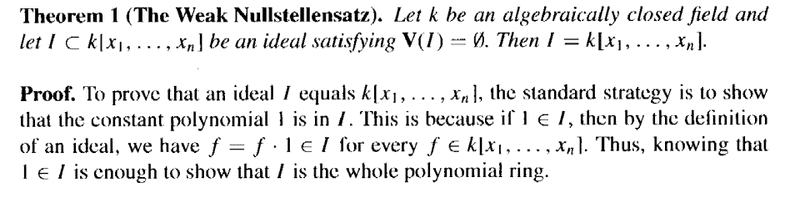# The Weak Nullstellensatz ... aspects of proof by Cox et al

Gold Member

## Homework Statement

I am reading the undergraduate introduction to algebraic geometry entitled "Ideals, Varieties and Algorithms: An introduction to Computational Algebraic Geometry and Commutative Algebra (Third Edition) by David Cox, John Little and Donal O'Shea ... ...

I am currently focused on Chapter 4, Section 1: Hilbert's Nullstellensatz ... ... and need help with the aspects of Cox et al's interesting proof of the Weak Nullstellensatz as outlined in Exercise 3 ...

Exercise 3 (Chapter 4, Section 1) reads as follows:As Exercise 3 refers to aspects of the proof of Theorem 1: The Weak Nullstellensatz, I am providing the first part of the proof of that Theorem as follows:My question is as follows:

How do we formulate and state a formal and rigorous proof of 3(a) ... ... that is a proof of the proposition that the coefficient ##c(a_1, a_2, \ ... \ ... \ , a_n )## of ##\tilde{x}^N_1## in ##f## is ##h_N (1, a_2, \ ... \ ... \ , a_n )## ... ...

## Homework Equations

[/B]
Relevant equations (such as the transformation from ##f_1## to ##\tilde{f}_1## are included in the attempt at a solution ...

## The Attempt at a Solution

[/B]
Being unable to formulate a rigorous and general proof I have studies an example case which indicates the proposition is likely to be true ... but not sure about formulating a general proof ... the worked example follows ...

Consider ##f_1 = 3 x_1^2 x_2^2 x_3 + 2 x_2^2 x_3^2##

The total degree of ##f_1## is ##N= 5##, determined by the first term, namely ##3 x_1^2 x_2^2 x_3## ... ...

Also note that ##h_N(x_1, x_2, x_3) = h_5(x_1, x_2, x_3) = 3 x_1^2 x_2^2 x_3##

and that ##h_5( 1, a_2, a_3) = 3 a_2^2 a_3##

... ... ...

Consider now the transformation f_1 \mapsto \tilde{f_1} given by:

##x_1 = \tilde{x}_1##

##x_2 = \tilde{x}_2 + a_2 \tilde{x}_1##

... ...

... ...

##x_n = \tilde{x}_n + a_n \tilde{x}_1##

Considering the above transformation, it is clear that the term ##3 x_1^2 x_2^2 x_3## will give rise the the coefficient of## \tilde{x}^N_1## ... ... so we examine this term under the transformation ... ...

... so then ...

##3 x_1^2 x_2^2 x_3 = 3 \tilde{x}_1^2 ( \tilde{x}_2^2 + 2 a_2 \tilde{x}_1 \tilde{x}_2 + a_2^2 \tilde{x}_1^2 ) ( \tilde{x}_3 + a_3 \tilde{x}_1 )##

##= ( 3 \tilde{x}_1^2 \tilde{x}_2^2 + 6 a_2 \tilde{x}_1^3 \tilde{x}_2 + 3 a_2^2 \tilde{x}_1^4 ) ( \tilde{x}_3 + a_3 \tilde{x}_1 )##

Clearly, the term involving ##\tilde{x}_1^N = \tilde{x}_1^5## will be

##3 a_2^2 a_3 \tilde{x}_1^5##

So we have that ##h_N( \tilde{x}_1, \tilde{x}_2, \tilde{x}_3 )## in ##\tilde{f}_1## is

##h_5( \tilde{x}_1, \tilde{x}_2, \tilde{x}_3 ) = 3 a_2^2 a_3 \tilde{x}_1^5##

so we have that

##h_5( 1, a_2, a_3 ) = 3 a_2^2 a_3##

as required ... ... BUT ...

... ... how do we formulate and state a formal proof of the general proposition that the coefficient ##c(a_1, a_2, \ ... \ ... \ , a_n )## of ## \tilde{x}^N_1## in ##f## is ##h_N (1, a_2, \ ... \ ... \ , a_n )## ... ... ?

Does the proof just describe the computation process in general terms ... ?

Hope someone can help ... ...

Help will be appreciated ...

Peter

#### Attachments

fresh_42
Mentor
2021 Award
My question is as follows:

How do we formulate and state a formal and rigorous proof of 3(a) ... ... that is a proof of the proposition that the coefficient ##c(a_1, a_2, \ ... \ ... \ , a_n )## of ##\tilde{x}^N_1## in ##f## is ##h_N (1, a_2, \ ... \ ... \ , a_n )## ... ...
I tried the same route as you. I wrote some general terms and quickly ended up with an example that was even easier than yours: only two variables ##x,y## but two terms, not one of total degree ##3.##
... ... how do we formulate and state a formal proof of the general proposition that the coefficient ##c(a_1, a_2, \ ... \ ... \ , a_n )## of ## \tilde{x}^N_1## in ##f## is ##h_N (1, a_2, \ ... \ ... \ , a_n )## ... ... ?
With a heck a lot of patience I guess ...
Does the proof just describe the computation process in general terms ... ?
Yes. If you really want to do it, I suggest to take an inductive process over the number of variables. Otherwise you'll have to be enormously careful not to get lost in indices.

Another approach could be to substitute the points ##a_i \; (i > 1)## at the very start. As long as you do not specify them, it makes no difference whether they are points or variables, which are anyway seen as coordinates of your variety. One has only to keep an eye on the highest monomials.

In general, you might have to change your view a bit from the pure algebraic point to a more geometric point, because that is what your textbook is about. The algebraic objects here mainly serve the role of a toolbox to analyse geometric varieties and to establish a kind of dictionary that translates one to the other and vice versa.

Gold Member
I tried the same route as you. I wrote some general terms and quickly ended up with an example that was even easier than yours: only two variables ##x,y## but two terms, not one of total degree ##3.##

With a heck a lot of patience I guess ...

Yes. If you really want to do it, I suggest to take an inductive process over the number of variables. Otherwise you'll have to be enormously careful not to get lost in indices.

Another approach could be to substitute the points ##a_i \; (i > 1)## at the very start. As long as you do not specify them, it makes no difference whether they are points or variables, which are anyway seen as coordinates of your variety. One has only to keep an eye on the highest monomials.

In general, you might have to change your view a bit from the pure algebraic point to a more geometric point, because that is what your textbook is about. The algebraic objects here mainly serve the role of a toolbox to analyse geometric varieties and to establish a kind of dictionary that translates one to the other and vice versa.